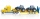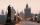Family 8

Father is 38 years old, daughter 12, son 14. How many years will father have as many years as his children together?

Result

x =  12

Solution:

38+x = (12+x) + (14+x)

x = 12

x = 12

Calculated by our simple equation calculator.

Leave us a comment of example and its solution (i.e. if it is still somewhat unclear...):

Showing 1 comment:Math student
I still have no idea how to you even got this because i haven't learned this yet and i don't even know why im searching it up please don't ask whyTo solve this verbal math problem are needed these knowledge from mathematics:

Do you have a linear equation or system of equations and looking for its solution? Or do you have quadratic equation?

Next similar examples:

1. How oldThe student who asked how many years he answered: "After 10 years I will be twice as old than as I was four years ago. How old is student?
2. SuzanSusan's age will be after 12 years four times as much as twelve years ago. How old is Susan now?
3. Bed timeTiffany was 5 years old; her week night bedtime grew by ¼ hour each year. If, at age 18, her curfew time is 11pm, what was her bed time when she was 5 years old?
4. InfirmaryTwo thirds of children from the infirmary went on a trip seventh went to bathe and 40 children remained in the gym. How many children were treated in the infirmary?
5. Forest nurseryIn the forest nursery after winter, they found that 1/10 stems died out of them. For them, they land 193 new spruces. How many spruces are in the forest nursery?
6. Tickets 3A total of 645 tickets were sold for the school play. They were either adult tickets or student tickets. There were 55 fewer student tickets sold than adult tickets. How many adult tickets were sold?
7. NormThree workers planted 3555 seedlings of tomatoes in one dey. First worked at the standard norm, the second planted 120 seedlings more and the third 135 seedlings more than the first worker. How many seedlings were standard norm?
8. Tractors5 tractors will plow the field for 8 hours. In 2 hours, 1 tractor broke down. How long do the remaining tractors complete the plow?
9. Unknown number 11That number increased by three equals three times itself?
10. Simple equationSolve the following simple equation: 2. (4x + 3) = 2-5. (1-x)
11. Equation 29Solve next equation: 2 ( 2x + 3 ) = 8 ( 1 - x) -5 ( x -2 )
12. Find xSolve: if 2(x-1)=14, then x= (solve an equation with one unknown)
13. Dropped sheetsThree consecutive sheets dropped from the book. The sum of the numbers on the pages of the dropped sheets is 273. What number has the last page of the dropped sheets?
14. TimeageSeven times of my age is 8 less than the largest two-digit number. How old I am?
15. Simple equationSolve for x: 3(x + 2) = x - 18
16. Negative in equation2x + 3 + 7x = – 24, what is the value of x?
17. Charles BridgeCharles Bridge was 15 times older than Frantův bridge from 1900 in 1931. How old is the Charles Bridge?